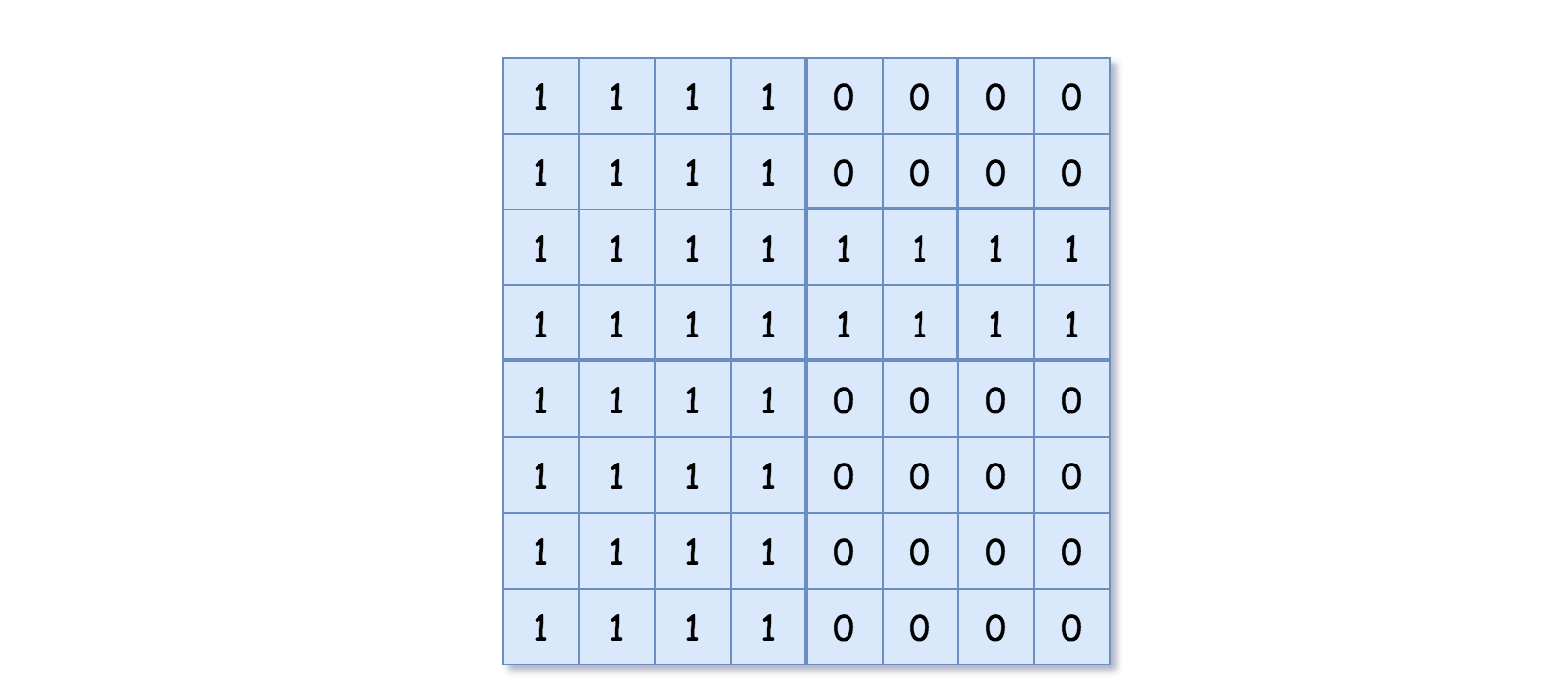Medium
Given a `n * n` matrix `grid` of `0's` and `1's` only. We want to represent the `grid` with a Quad-Tree.
Return the root of the Quad-Tree representing the `grid`.
Notice that you can assign the value of a node to True or False when `isLeaf` is False, and both are accepted in the answer.
A Quad-Tree is a tree data structure in which each internal node has exactly four children. Besides, each node has two attributes:
• `val`: True if the node represents a grid of 1's or False if the node represents a grid of 0's.
• `isLeaf`: True if the node is leaf node on the tree or False if the node has the four children.
class Node {
public boolean val;
public boolean isLeaf;
public Node topLeft;
public Node topRight;
public Node bottomLeft;
public Node bottomRight;
}
We can construct a Quad-Tree from a two-dimensional area using the following steps:
1. 1.
If the current grid has the same value (i.e all `1's` or all `0's`) set `isLeaf` True and set `val` to the value of the grid and set the four children to Null and stop.
2. 2.
If the current grid has different values, set `isLeaf` to False and set `val` to any value and divide the current grid into four sub-grids as shown in the photo.
3. 3.
Recurse for each of the children with the proper sub-grid.If you want to know more about the Quad-Tree, you can refer to the wiki.
The output represents the serialized format of a Quad-Tree using level order traversal, where `null` signifies a path terminator where no node exists below.
It is very similar to the serialization of the binary tree. The only difference is that the node is represented as a list `[isLeaf, val]`.
If the value of `isLeaf` or `val` is True we represent it as 1 in the list `[isLeaf, val]` and if the value of `isLeaf` or `val` is False we represent it as 0.
Example 1:Input: grid = [[0,1],[1,0]]
Output: [[0,1],[1,0],[1,1],[1,1],[1,0]]
Explanation: The explanation of this example is shown below:
Notice that 0 represnts False and 1 represents True in the photo representing the Quad-Tree.
Example 2:Input: grid = [[1,1,1,1,0,0,0,0],[1,1,1,1,0,0,0,0],[1,1,1,1,1,1,1,1],[1,1,1,1,1,1,1,1],[1,1,1,1,0,0,0,0],[1,1,1,1,0,0,0,0],[1,1,1,1,0,0,0,0],[1,1,1,1,0,0,0,0]]
Output: [[0,1],[1,1],[0,1],[1,1],[1,0],null,null,null,null,[1,0],[1,0],[1,1],[1,1]]
Explanation: All values in the grid are not the same. We divide the grid into four sub-grids.
The topLeft, bottomLeft and bottomRight each has the same value.
The topRight have different values so we divide it into 4 sub-grids where each has the same value.
Explanation is shown in the photo below:
Constraints:
• `n == grid.length == grid[i].length`
• `n == 2x` where `0 <= x <= 6`

### 解題

/**
* Definition for a QuadTree node.
* type Node struct {
* Val bool
* IsLeaf bool
* TopLeft *Node
* TopRight *Node
* BottomLeft *Node
* BottomRight *Node
* }
*/
func construct(grid [][]int) *Node {
return helper(grid, 0, 0, len(grid))
}
func helper(grid [][]int, x, y, w int) *Node {
if allSame(grid, x, y, w) {
n := &Node{ IsLeaf: true }
if grid[x][y] == 1 {
n.Val = true
} else {
n.Val = false
}
return n
}
return &Node{
Val : true,
IsLeaf : false,
TopLeft: helper(grid, x, y, w/2),
TopRight: helper(grid, x, y + w/2, w/2),
BottomLeft: helper(grid, x + w/2, y, w/2),
BottomRight: helper(grid, x + w/2, y + w/2, w/2)}
}
func allSame(grid [][]int, x, y, w int) bool {
val := grid[x][y]
for i:=x; i<x+w; i++ {
for j:=y; j<y+w; j++ {
if grid[i][j] != val {
return false
}
}
}
return true
}